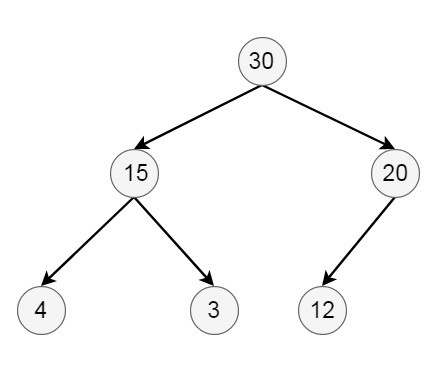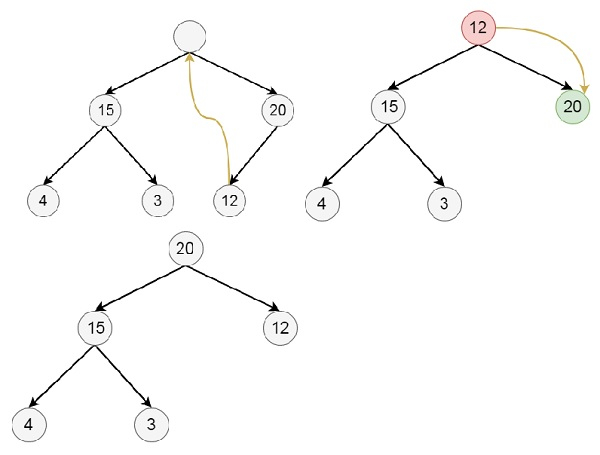# Deletion from a Max Heap in Data Structure

Here we will see how to delete elements from binary max heap data structures. Suppose the initial tree is like below −## Deletion Algorithm

delete(heap, n) −
Begin
if heap is empty, then exit
else
item := heap
last := heap[n]
n := n – 1
for i := 1, j := 2, j <= n, set i := j and j := j * 2, do
if j < n, then
if heap[j] < heap[j + 1], then j := j + 1
end if
if last >= heap[j], then break
heap[i] := heap[j]
done
end if
heap[i] := last
End

## Example

Suppose we want to delete 30 from the final heap −